Transformer 是谷歌大脑在 2017 年底发表的论文 attention is all you need 中所提出的 seq2seq 模型。现在已经取得了大范围的应用和扩展，而 BERT 就是从 Transformer 中衍生出来的预训练语言模型

# Transformer 直观认识

Transformer 和 LSTM 的最大区别，就是 LSTM 的训练是迭代的、串行的，必须要等当前字处理完，才可以处理下一个字。而 Transformer 的训练时并行的，即所有是同时训练的，这样就大大增加了计算效率。Transformer 使用了位置嵌入 (Positional Encoding) 来理解语言的顺序，使用自注意力机制（Self Attention Mechanism）和全连接层进行计算，这些后面会讲到

Transformer 模型主要分为两大部分，分别是 Encoder 和 DecoderEncoder 负责把输入（语言序列）隐射成隐藏层（下图中第 2 步用九宫格代表的部分），然后解码器再把隐藏层映射为自然语言序列。例如下图机器翻译的例子（Decoder 输出的时候，是通过 N 层 Decoder Layer 才输出一个 token，并不是通过一层 Decoder Layer 就输出一个 token）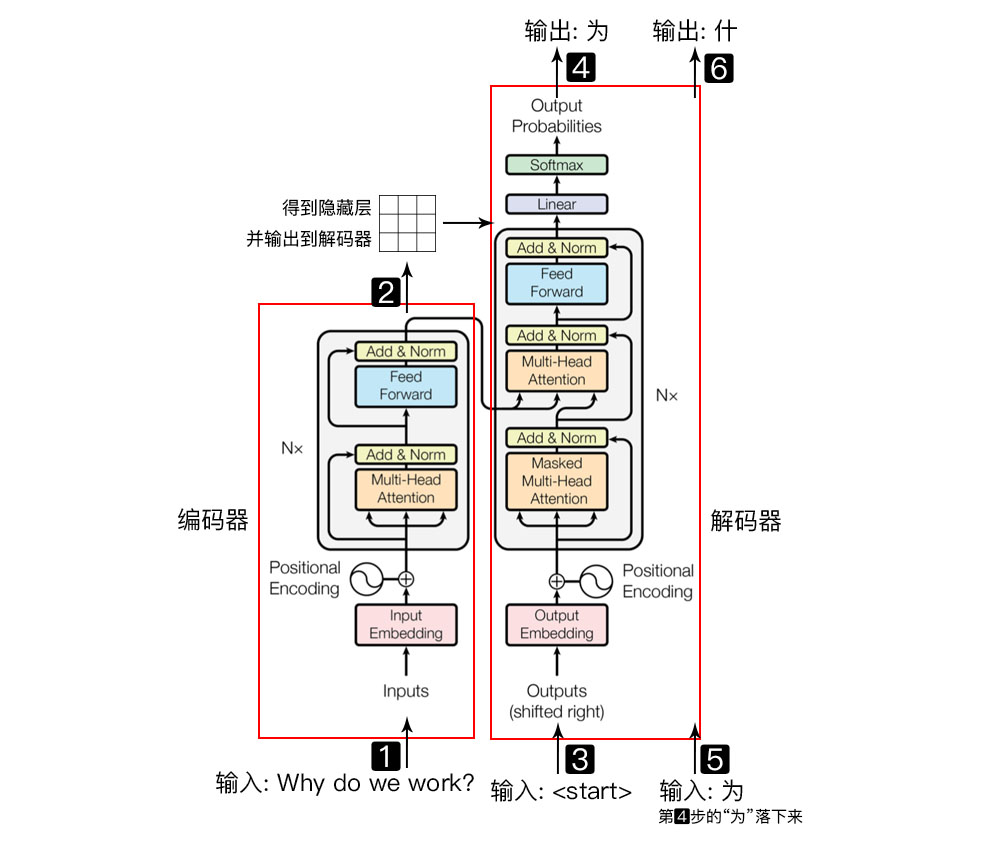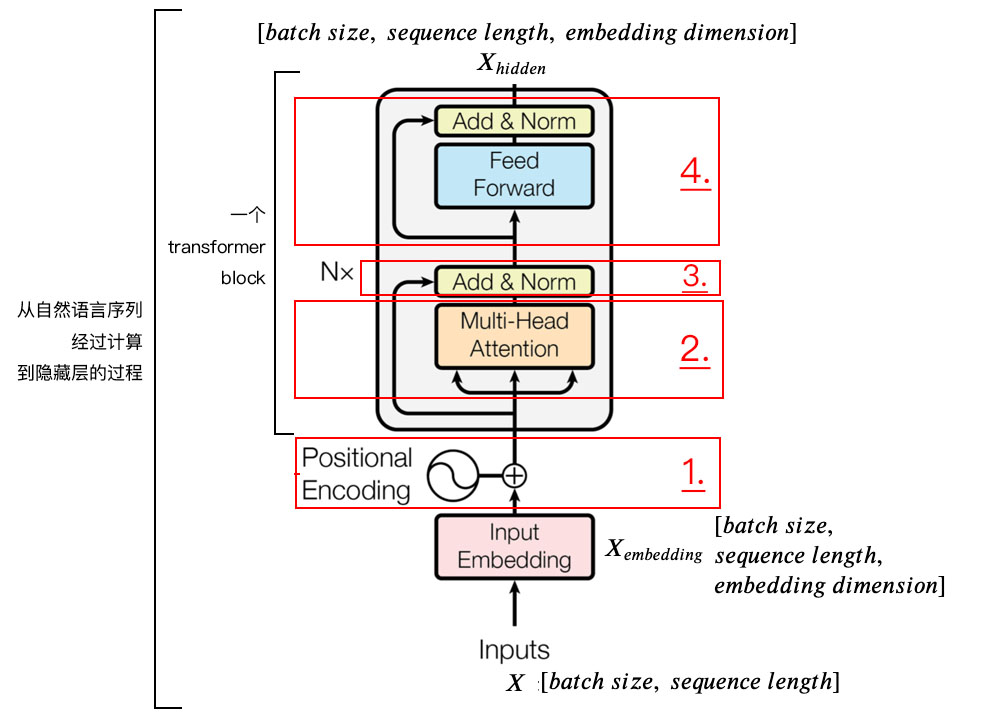# 1. Positional Encoding

$PE{(pos,2i)} = \sin(pos / 10000^{2i/d_{\text{model}}}) \\ PE{(pos,2i+1)} = \cos(pos / 10000^{2i/d_{\text{model}}})$

import numpy as np
import matplotlib.pyplot as plt
import seaborn as sns
import math

def get_positional_encoding(max_seq_len, embed_dim):
# 初始化一个positional encoding
# embed_dim: 字嵌入的维度
# max_seq_len: 最大的序列长度
positional_encoding = np.array([
[pos / np.power(10000, 2 * i / embed_dim) for i in range(embed_dim)]
if pos != 0 else np.zeros(embed_dim) for pos in range(max_seq_len)])

positional_encoding[1:, 0::2] = np.sin(positional_encoding[1:, 0::2])  # dim 2i 偶数
positional_encoding[1:, 1::2] = np.cos(positional_encoding[1:, 1::2])  # dim 2i+1 奇数
return positional_encoding

positional_encoding = get_positional_encoding(max_seq_len=100, embed_dim=16)
plt.figure(figsize=(10,10))
sns.heatmap(positional_encoding)
plt.title("Sinusoidal Function")
plt.xlabel("hidden dimension")
plt.ylabel("sequence length")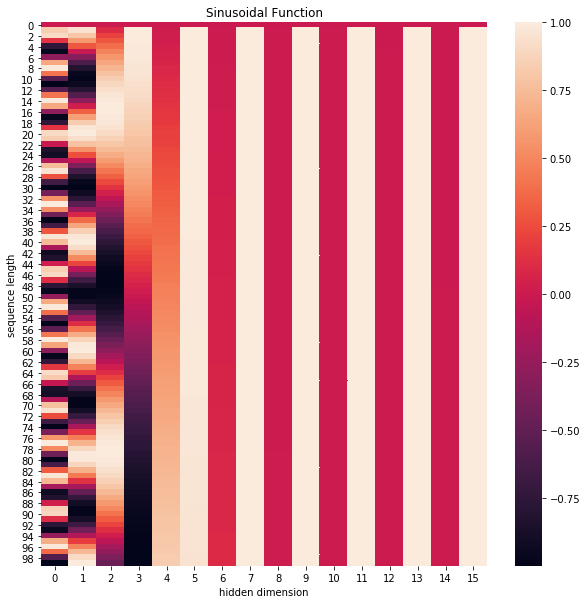plt.figure(figsize=(8, 5))
plt.plot(positional_encoding[1:, 1], label="dimension 1")
plt.plot(positional_encoding[1:, 2], label="dimension 2")
plt.plot(positional_encoding[1:, 3], label="dimension 3")
plt.legend()
plt.xlabel("Sequence length")
plt.ylabel("Period of Positional Encoding")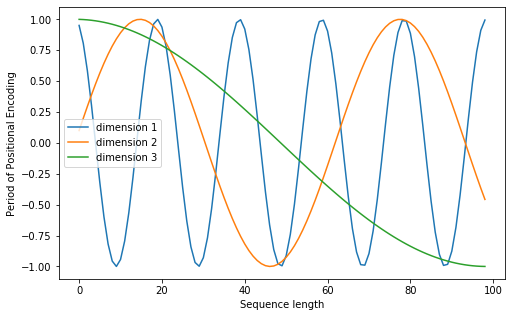# 2. Self Attention Mechanism[0, 4, 2]
[1, 0, 2] x [1, 4, 3] = [2, 4, 4]
[1, 0, 1]之后还需要将得到的值经过 softmax，使得它们的和为 1（见下图）

 softmax([2, 4, 4]) = [0.0, 0.5, 0.5]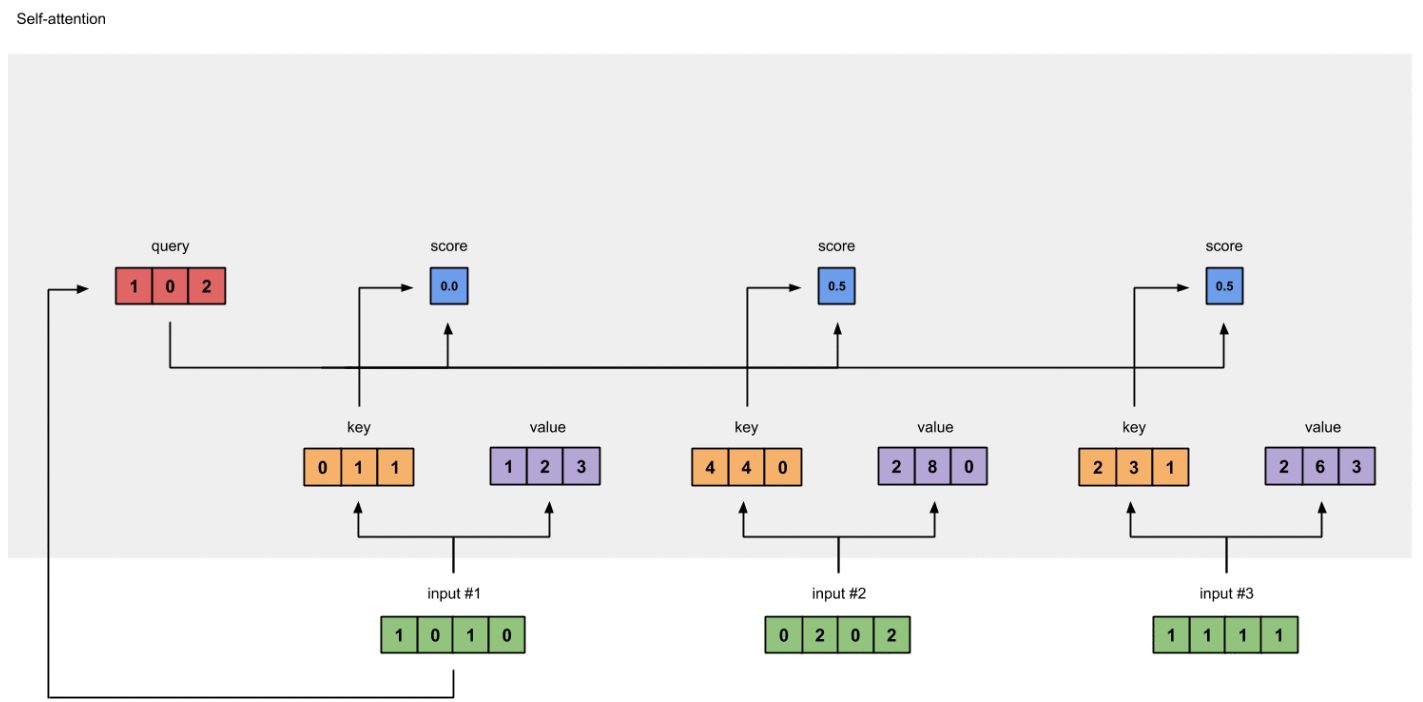0.0 * [1, 2, 3] = [0.0, 0.0, 0.0]
0.5 * [2, 8, 0] = [1.0, 4.0, 0.0]
0.5 * [2, 6, 3] = [1.0, 3.0, 1.5][0.0, 0.0, 0.0]
+ [1.0, 4.0, 0.0]
+ [1.0, 3.0, 1.5]
-----------------
= [2.0, 7.0, 1.5]## 2.1 矩阵计算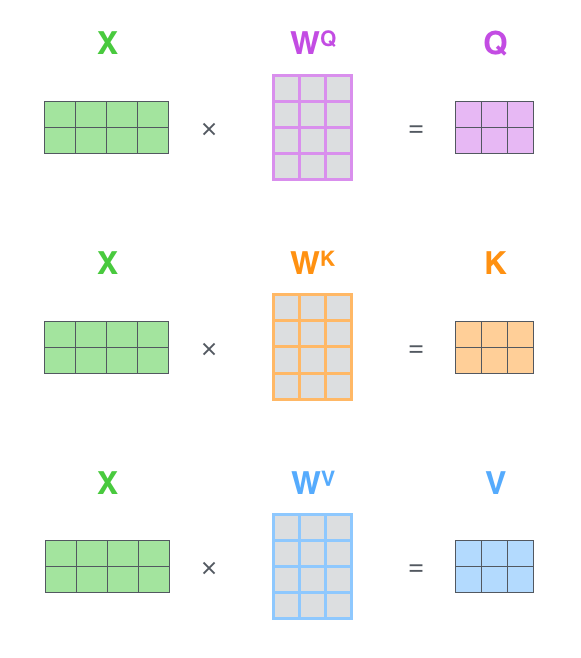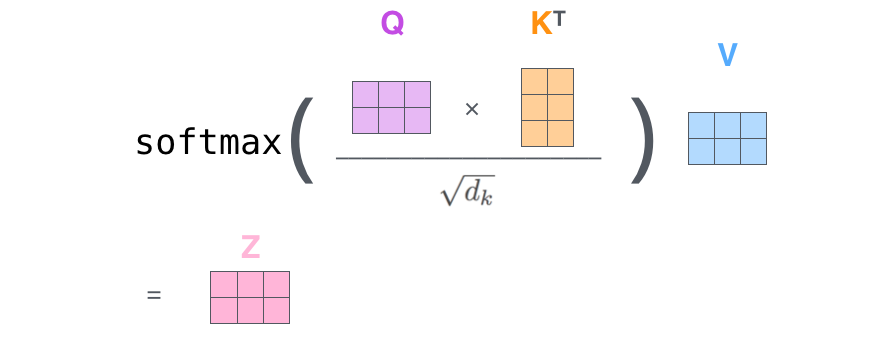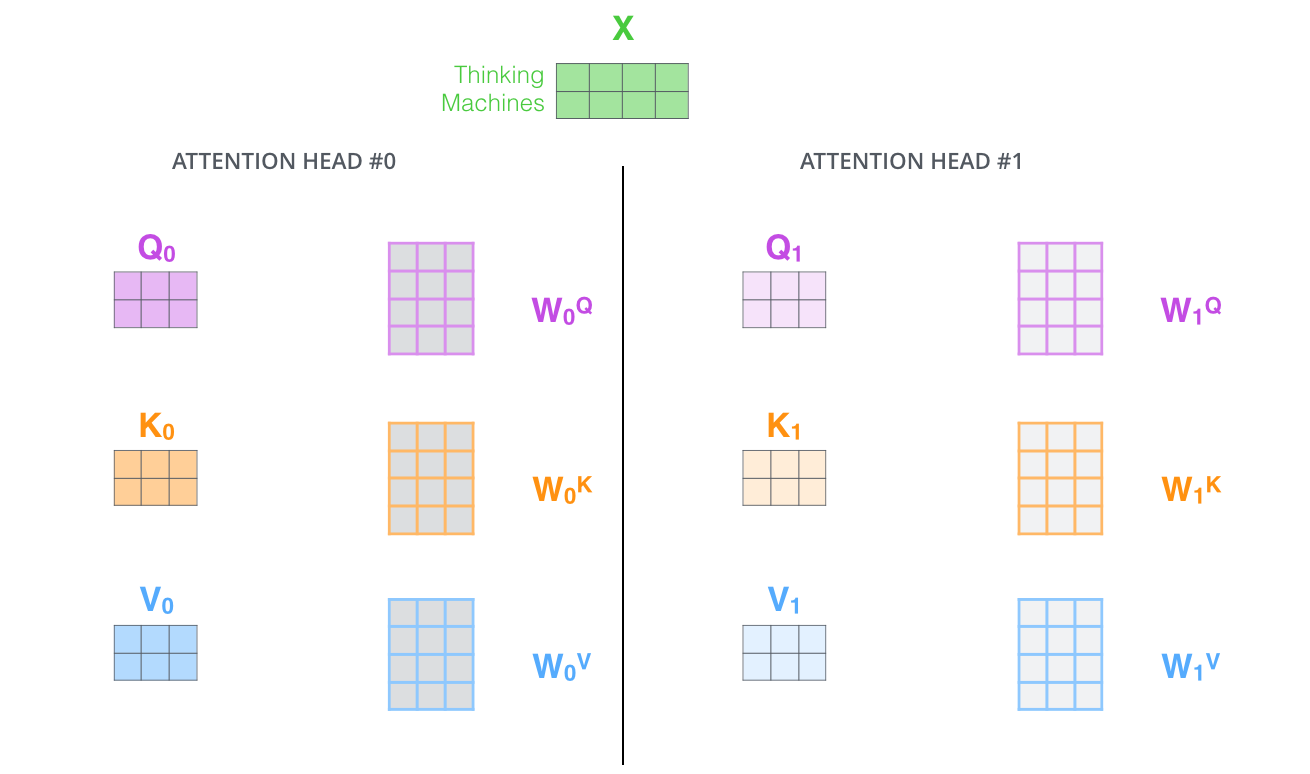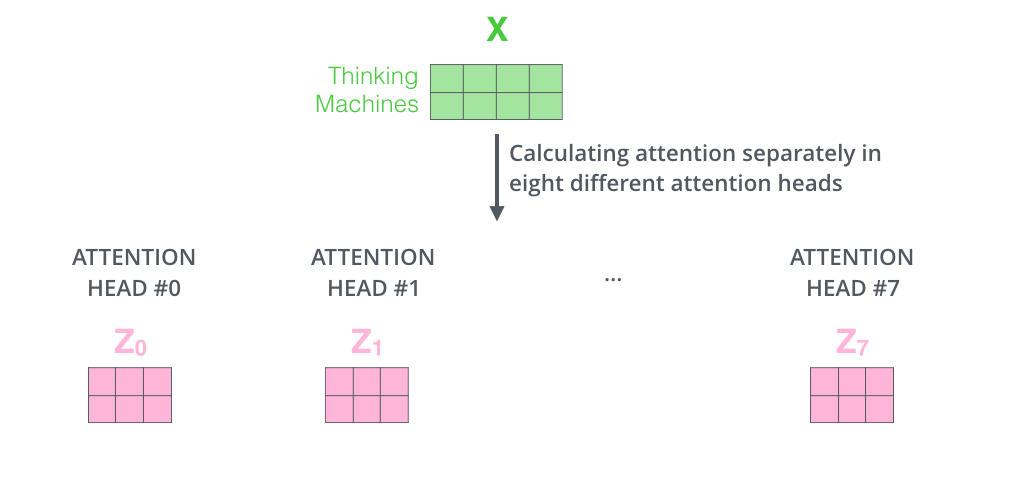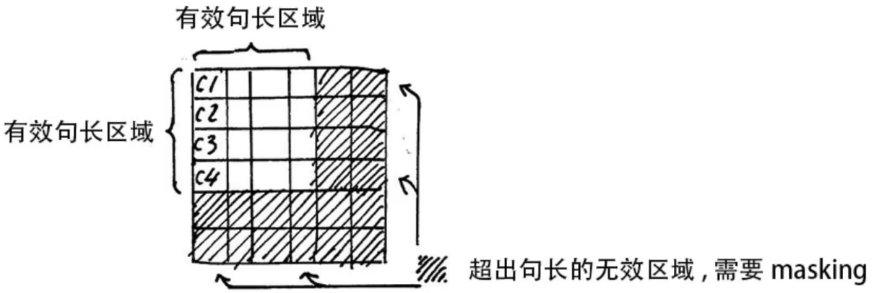\begin{aligned} &Z_{\text {illegal }}=Z_{\text {illegal }}+\text { bias }_{\text {illegal }} \\ &\text { bias }_{\text {illegal }} \rightarrow-\infty \end{aligned}

# 3. 残差连接和 Layer Normalization

## 3.1 残差连接

$X_{embedding} + \text{Self-Attention}(Q, \ K, \ V)$

## 3.2 Layer Normalization

Layer Normalization 的作用是把神经网络中隐藏层归一为标准正态分布，也就是 i.i.d 独立同分布，以起到加快训练速度，加速收敛的作用

$$\mu_{j}=\frac{1}{m} \sum_{i=1}^{m} x_{i j}$$

$$\sigma_{j}^{2}=\frac{1}{m} \sum_{i=1}^{m}\left(x_{i j}-\mu_{j}\right)^{2}$$

$$\text { Layer } N o r m(x)=\frac{x_{i j}-\mu_{j}}{\sqrt{\sigma_{j}^{2}+\epsilon}}$$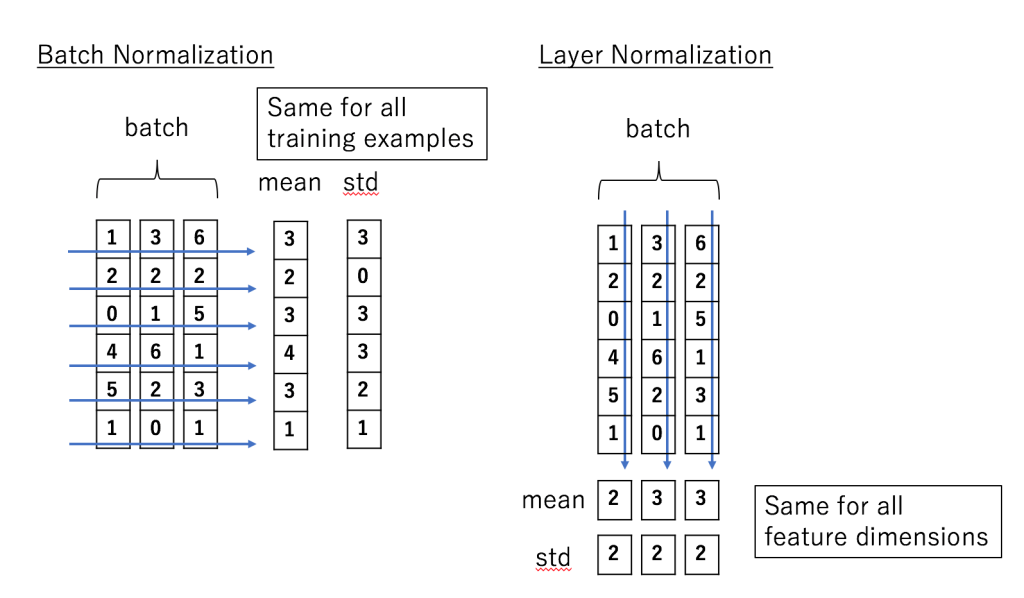# 4. Transformer Encoder 整体结构

1). 字向量与位置编码
$$X= Embedding-Lookup (X)+ Positional-Encoding$$
2). 自注意力机制
\begin{aligned} Q &=\operatorname{Linear}_{q}(X)=X W_{Q} \\ K &=\operatorname{Linear}_{k}(X)=X W_{K} \\ V &=\operatorname{Linear}_{v}(X)=X W_{V} \\ X_{\text {attention }} &=\operatorname{Self}-\text { Attention }(Q, K, V) \end{aligned}
3). self-attention 残差连接与 Layer Normalization
$$\begin{gathered} X_{\text {attention }}=X+X_{\text {attention }} \\ X_{\text {attention }}=\operatorname{LayerNorm}\left(X_{\text {attention }}\right) \end{gathered}$$
4). 下面进行 Encoder block 结构图中的第 4 部分，也就是 FeedForward，其实就是两层线性映射并 用激活函数激活，比如说 ReLU
$$X_{\text {hidden }}=\operatorname{Linear}\left(\operatorname{ReLU}\left(\operatorname{Linear}\left(X_{\text {attention }}\right)\right)\right)$$
5). FeedForward 残差连接与 Layer Normalization
$$\begin{gathered} X_{\text {hidden }}=X_{\text {attention }}+X_{\text {hidden }} \\ X_{\text {hidden }}=\operatorname{LayerNorm}\left(X_{\text {hidden }}\right) \end{gathered}$$

$$X_{\text {hidden }} \in \mathbb{R}^{\text {batch_size } * \text { seq_len. *embed_dim }}$$

# 5. Transformer Decoder 整体结构

• FeedForward Network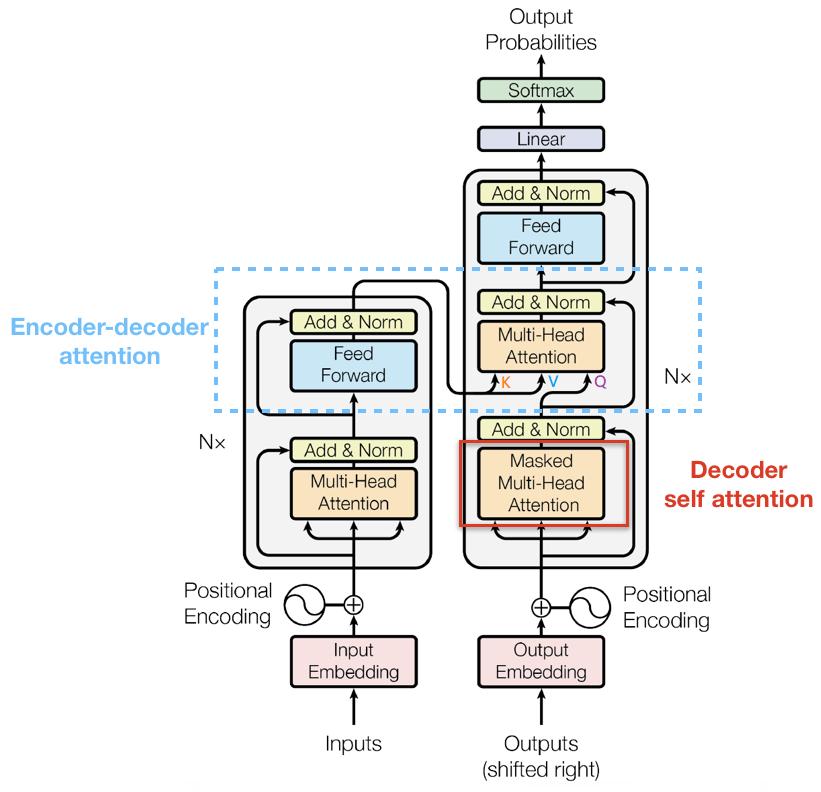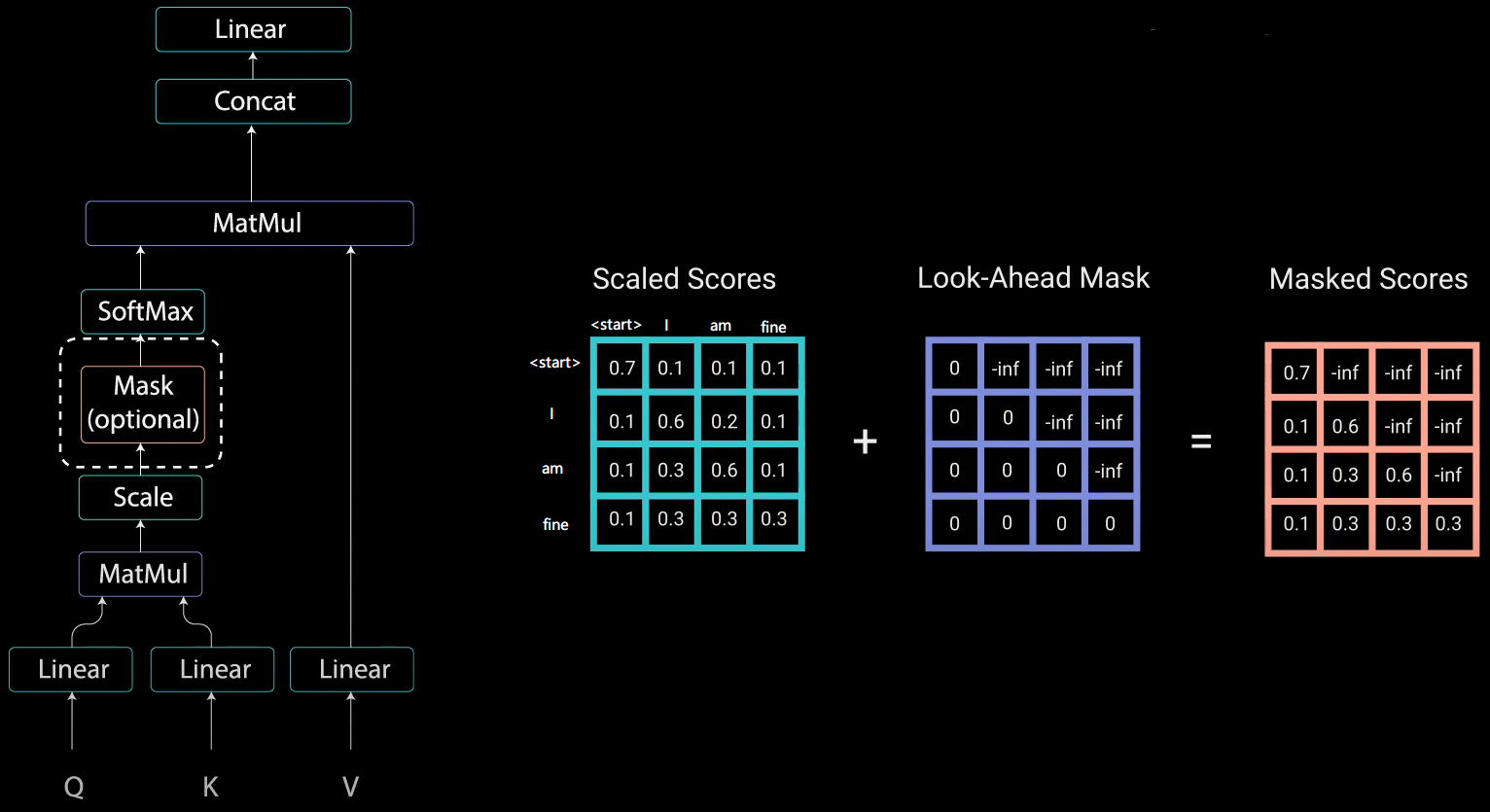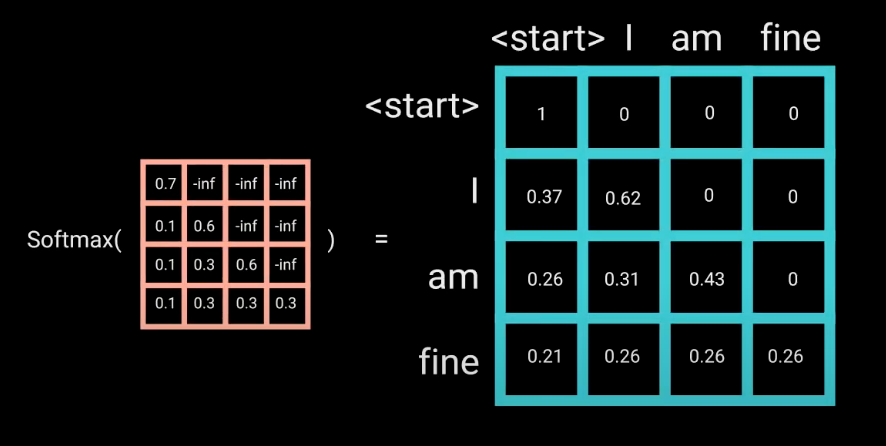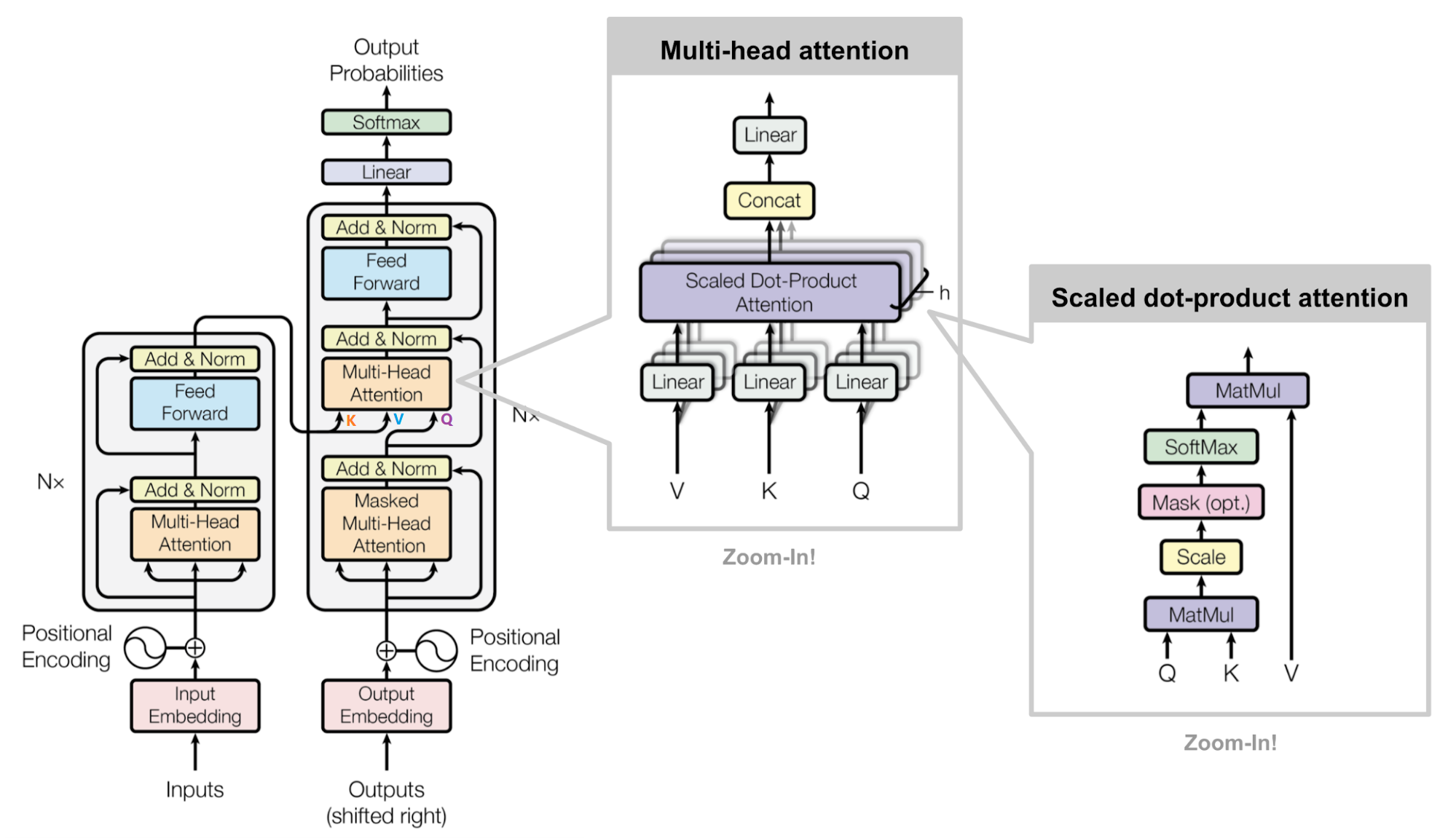# 6. 总结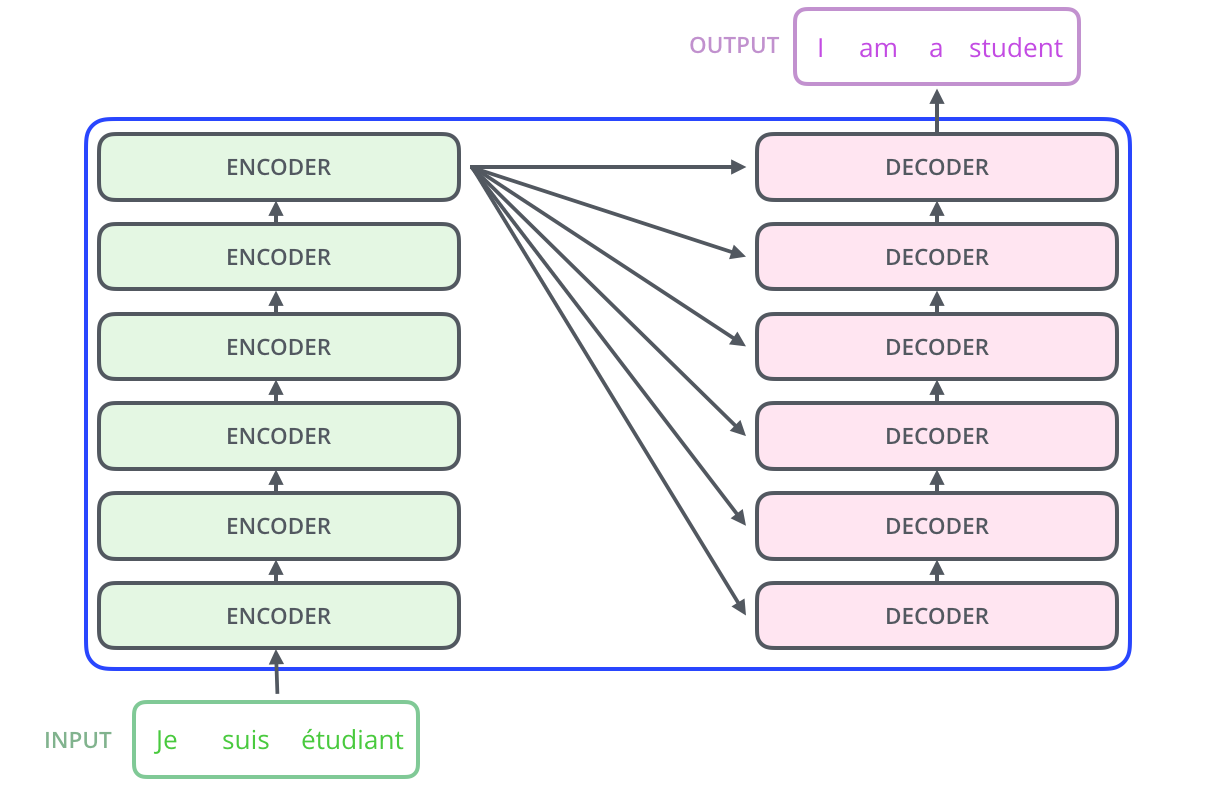## 6.2 Transformer 相比于 RNN/LSTM，有什么优势？为什么？

1. RNN 系列的模型，无法并行计算，因为 T 时刻的计算依赖 T-1 时刻的隐层计算结果，而 T-1 时刻的计算依赖 T-2 时刻的隐层计算结果
2. Transformer 的特征抽取能力比 RNN 系列的模型要好

# 7. 参考文章

••